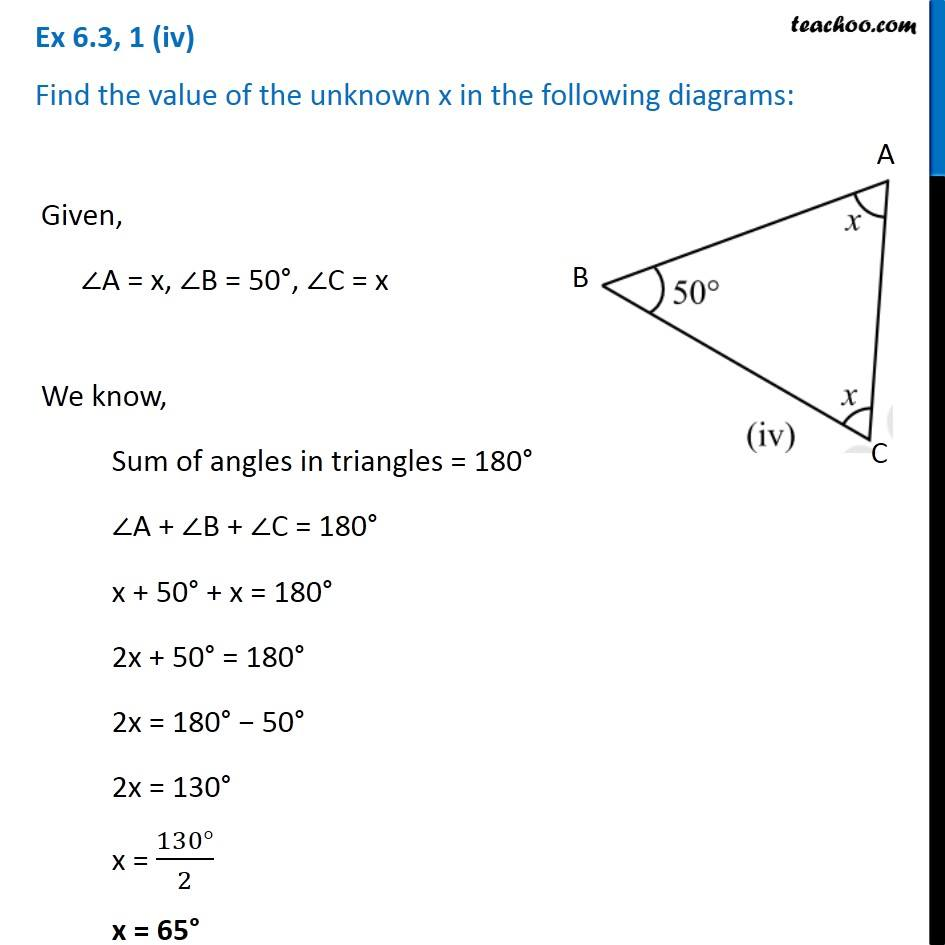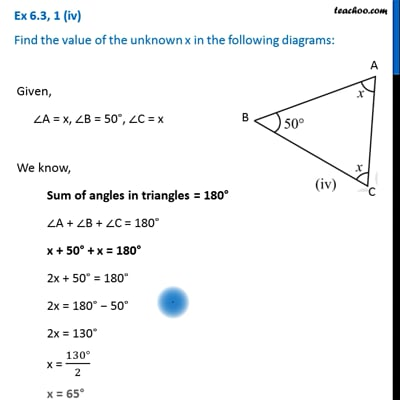Ex 6.3

Chapter 6 Class 7 Triangle and its Properties
Serial order wiseThis video is only available for Teachoo black users

Learn in your speed, with individual attention - Teachoo Maths 1-on-1 Class

### Transcript

Ex 6.3, 1 (iv) Find the value of the unknown x in the following diagrams: Given, ∠A = x, ∠B = 50°, ∠C = x We know, Sum of angles in triangles = 180° ∠A + ∠B + ∠C = 180° x + 50° + x = 180° 2x + 50° = 180° 2x = 180° − 50° 2x = 130° x = (130°)/2 x = 65°